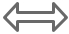# Converting inches to cm and vice versa

Inches (In)Centimeters (Cm)

## How this script works

When we enter any number at inches textbox ,we used onblur and onkeyup events to run the functions written to convert inches to cm `in_to_cm()`.

Similarly when we enter any data at centimetre textbox, it fires the function written to convert CM to Inches `cm_to_in()` by using onblur and onkeyup events.

Inside the functions we used simple mathematical conversion formulas to arrive at the result and display the same using getElementById()
``````<script language=JavaScript>
<!--
function in_to_cm()
{
var v_in=document.getElementById("t_in").value;
var v_cm=v_in*2.54;
document.getElementById("t_cm").value=v_cm;
}

function cm_to_in()
{
var v_cm=document.getElementById("t_cm").value;
var v_in=v_cm*0.39370079;
document.getElementById("t_in").value=v_in;
}
//-->
</script>``````## Subscribe

* indicates required
Subscribe to plus2netplus2net.com

Post your comments , suggestion , error , requirements etc here .